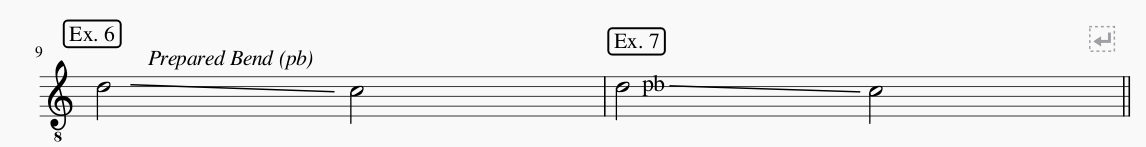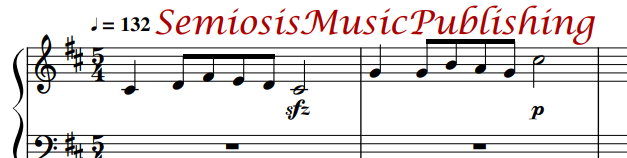Bends.

Bend. The notation for a bend is a straight line between the initial and terminal notes, annotated with either the expression Bend or in-line with the abbreviation b. When first introduced and later followed by abbreviation b, the annotation is Bend (b). Bend implies that the terminal note is sutained for its duration. It provides no further information about its release. When the abbreviation b appears in-line, it appears at the beginning of the line.

Example 1 illustrates how to introduce Bend (b) in a composition. The guitarist interprets the straight line between C and D to mean a bend from C to D and that b is an abbreviation for later bends in the score. Example 2 is an example of an in-line abbeviation to bend C to D.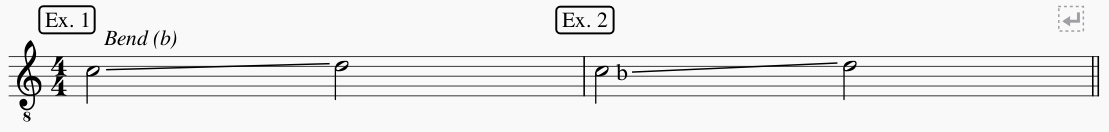When there isn't enough space between the initial and terminal notes to render a line, or where a grace note provides a line crossing a note, the notation for a a bend is b.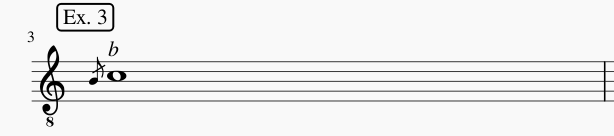Release. The notation for a release is a straight line between the sustained and terminal notes following a bend annotated either with the expression Release or in-line with the abbreviation r. When first introduced and later followed by abbreviation r, the annotation is Release (r). When the abbreviation r appears in-line, it appears at the end of the line.

Example 4 illustrates how to introduce Release (r) in a composition. The guitarist interprets the straight line between D and C, following the bend from C to D to mean release the bend from D to C and that r is an abbreviation for later bends in the score. Example 5 is an example of an in-line abbeviation to release D to C.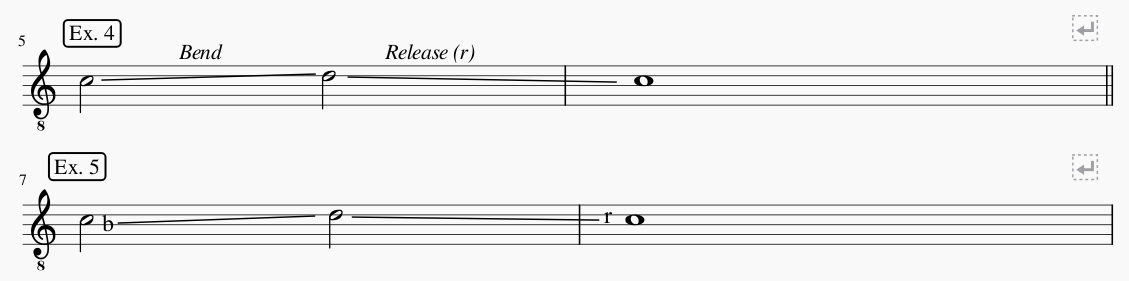Prepared Bend. The notation for a prepared bend is a straight line between the initial and terminal notes, annotated with either the expression Prepared Bend or in-line with the abbreviation pb. When first introduced and later followed by abbreviation pb, the annotation is Prepared Bend (pb).

Example 6 illustrates how to introduce Prepared Bend (pb) in a composition. The guitarist interprets the straight line between D and C to mean a release from D to C with no pre-existing bend to release and that pb is an abbreviation for later bends in the score. Example 7 is an example of an in-line abbeviation to of a prepare bend from D to C.